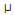# Electronics - Resistance and Power - Discussion

### Discussion :: Resistance and Power - General Questions (Q.No.28)

28.

How many ohms of resistance allows a current of 720A to flow when 3.6 kV is applied?

 [A]. 200 n[B]. 5 k[C]. 200 k[D]. 5 MExplanation:

No answer description available for this question.

 Karthik said: (Apr 9, 2012) R=V/I R=3600/0.000720 R=5000000=5MOhms